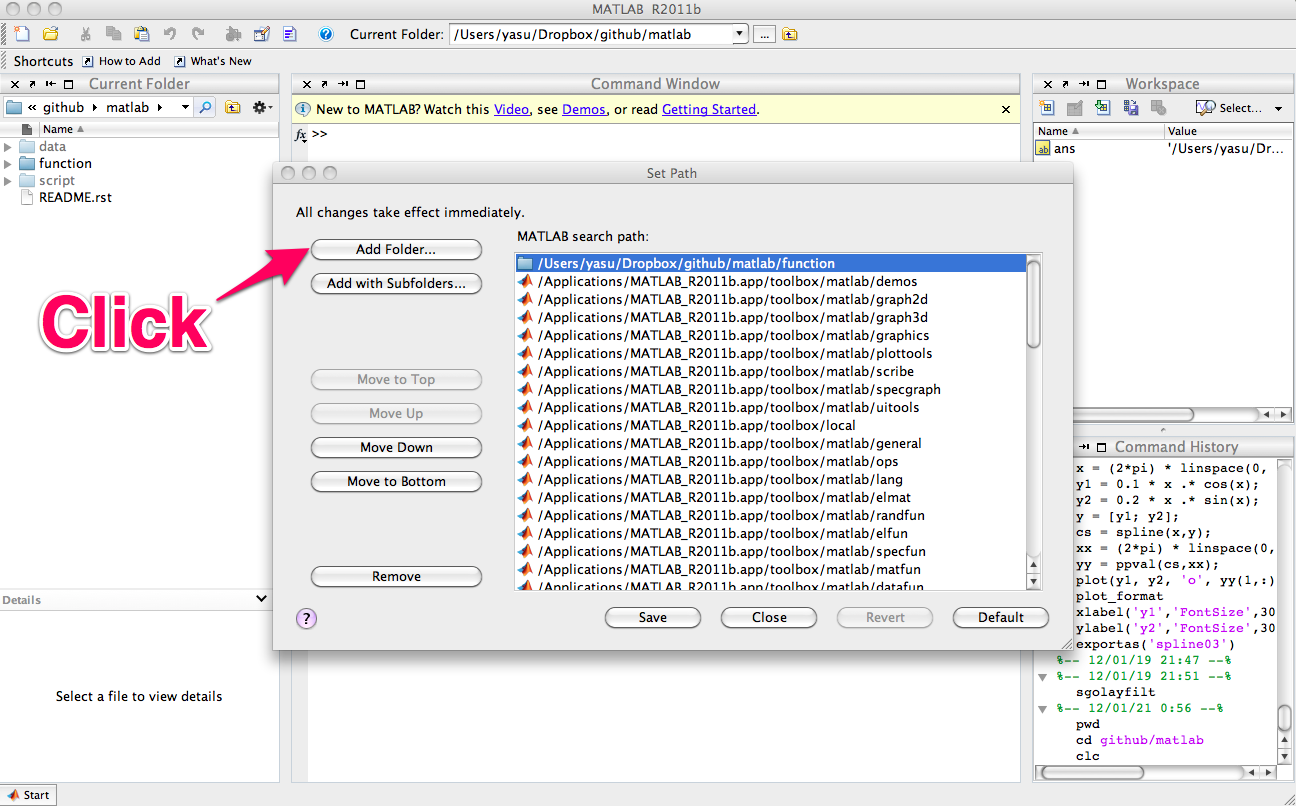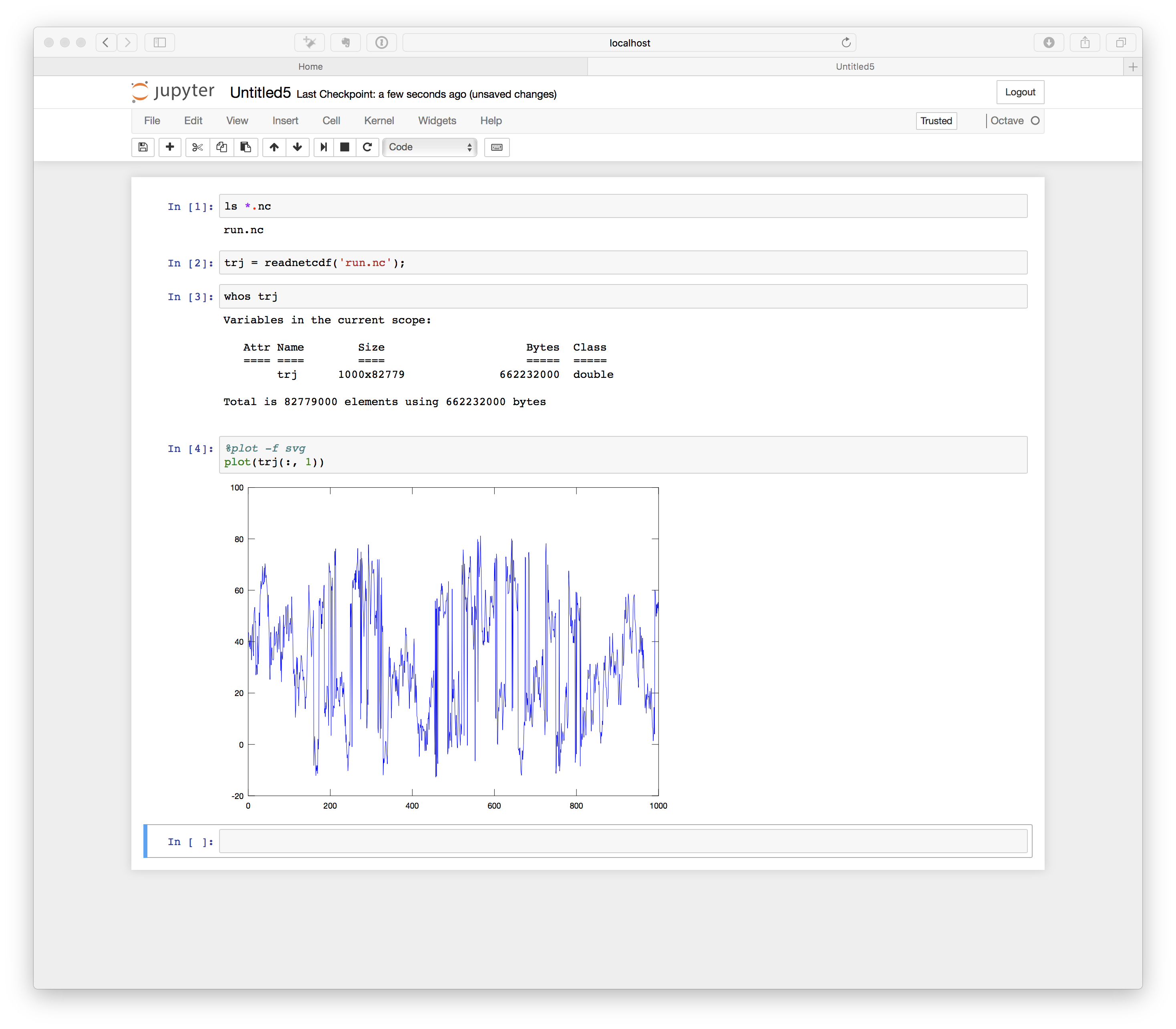# Introduction¶

## What is MDToolbox?¶

MDToolbox is a MATLAB/Octave toolbox for statistical analysis of molecular dynamics (MD) simulation data of biomolecules. It consists of a collection of functions covering the following types of scientific computations:

• I/O for trajectory, coordinate, and topology files used for MD simulation
• Least-squares fitting of structures
• Potential mean force (PMF) or free energy profile from scattered data
• Statistical estimates (WHAM and MBAR methods) from biased data
• Dimensional reductions (Principal Component Analysis, and others)
• Elastic network models (Gaussian and Anisotropic network models)
• Utility functions, such as atom selections

MDToolbox is developed on GitHub. Freely available under the BSD license.

## Requirements¶

MDToolbox is developed and tested on MATLAB R2013a and later versions.

We are also testing the toolbox on GNU Octave version 3.8.2. As far as we have checked, most of functions should work on Octave version 3.8.2 or laters.

Zip arichive or tarball of the latest version is available from GitHub, or the repository can be directly cloned from GitHub by using git,

```\$ git clone https://github.com/ymatsunaga/mdtoolbox.git
```

## Installation for MATLAB¶

For personal installation, the personal startup file may be found at `~/matlab/startup.m`. If it does not exist, create one. Add the following line to `startup.m` with full path to the directory of MDToolbox m-files,

```addpath('/path/to/mdtoolbox/mdtoolbox/')
```

For system-wide installation, call `pathtool` command in MATLAB and add the directory to the user’s MATLAB search path (root permission is required to save the path),## Installation for Octave¶

For personal installation, the personal startup file may be found at `~/octaverc`. If it does not exist, create one. Add the following line to `~/octaverc` with full path to the directory of MDToolbox m-files,

```addpath('/path/to/mdtoolbox/mdtoolbox/')
```

To use I/O functions for NetCDF files (e.g., AMBER NetCDF trajectory), netcdf package needs to be installed in Octave.

In addition to the m-files, MEX-files are prepared for core functions to accelerate the performance. We strongly recommend to use these MEX-files for reasonable performance in MATLAB/Octave. To use MEX-files, the user needs to compile the files in advance. For the compilation, use `make.m` script in MATLAB/Octave:

```>> cd /path/to/mdtoolbox/
>> make
```

Warnings during the compilation can be safely ignored.

On Linux platforms, OpenMP option can be enabled for further performance by parallel computation (multithreading),

```>> make('openmp')
```

For parallel run, make sure to set your environment variable (`OMP_NUM_THREADS`) before starting up MATLAB/Octave. For example, if you want to use 8 threads(=CPU cores) parallelization, the variable should be set from the shell prompt as follows:

```# for sh/bash/zsh
# for csh/tcsh
```

## Docker image for MDToolbox¶

A docker image for MDToolbox is avaiable here. In this docker image, you can use Octave already configured for use with MDToolbox (downloading MDtoolbox, path setup, MEX file compiling, etc are already completed).

By just running a docker command, you can immediately use MDToolbox (without GUI),

```\$ docker run -it --rm -v \$(pwd):/home/jovyan/work ymatsunaga/octave octave
```

Or you can use Octave + MDToolbox within Jupyter notebook (with GUI),

```\$ docker run --rm -p 8888:8888 -v \$(pwd):/home/jovyan/work ymatsunaga/octave
```For details of the usage, please see our docker image site.

## Summary of main functions¶

Representative functions of MDToolbox are summarized in the tables below. For detail of each function, use `help` command in MATLAB. For example, usage of `readdcd()` function can be obtained as follows:

```>> help readpdb
read xplor or charmm (namd) format dcd file

% Syntax
# [trj, box] = readdcd(filename, index_atom);

% Description
The XYZ coordinates of atoms are read into 'trj' variable
which has 'nstep' rows and '3*natom' columns.
Each row of 'trj' has the XYZ coordinates of atoms in order
[x(1) y(1) z(1) x(2) y(2) z(2) ... x(natom) y(natom) z(natom)].

* filename   - input dcd trajectory filename
* index_atom - index or logical index for specifying atoms to be read
* trj        - trajectory [nstep x natom3 double]
* box        - box size [nstep x 3 double]
[structure]

% Example

writedcd

% References for dcd format
MolFile Plugin http://www.ks.uiuc.edu/Research/vmd/plugins/molfile/dcdplugin.html
CafeMol Manual http://www.cafemol.org/doc.php
EGO_VIII Manual http://www.lrz.de/~heller/ego/manual/node93.html
```

Inuput/Output

name description
writepdb write Protein Data Bank (PDB) file
writeambercrd write amber coordinate/restart file
writemdcrd write amber ascii-format trajectory format file
writenetcdf write amber netcdf file
writedcd write xplor or charmm (namd) format dcd file
writenamdbin write namd restart (namdbin) file
writegro write gromacs gro (Gromos87 format) file
writedx write dx (opendx) format file

Geometry calculations (fitting of structures, distance, angles, dihedrals, etc)

name description
superimpose least-squares fitting of structures
meanstructure calculate average structure by iterative superimposing
decenter remove the center of mass from coordinates or velocities
orient orient molecule using the principal axes of inertia
searchrange finds all the atoms within cutoff distance from given atoms
calcbond calculate distance from the Cartesian coordinates of two atoms
calcangle calculate angle from the Cartesian coordinates of three atoms
calcdihedral calculate dihedral angle from the Cartesian coordinates of four atoms
calcpairlist make a pairlist by searching pairs within a cutoff distance
calcqscore calculate Q-score (fraction of native contacts) from given heavy atom coordinates

Statistics (WHAM, MBAR, clustering, etc)

name description
wham Weighted Histogram Analysis method (WHAM)
ptwham Parallel tempering WHAM (PTWHAM)
mbar multi-state Bennett Acceptrance Ratio Method (MBAR)
mbarpmf evaluate PMF from the result of MBAR
calcpmf calculate 1D potential of mean force from scattered 1D-data (using kernel density estimator)
calcpmf2d calculate 2D potential of mean force from scattered 2D-data (using kernel density estimator)
calcpca peform principal component analysis (PCA)
calctica perform time-structure based Independent Component Analysis (tICA)
clusterkcenters clustering by K-centers
clusterhybrid Hybrid clustering by using K-centers and K-medoids
clusterkmeans clustering by K-means

Anisotropic Network Model

name description
anm calculate normal modes and anisotropic fluctuations by using Anisotropic Network Model.
anmsparse calculate normal modes of ANM using sparse-matrix for reducing memory size
anmsym calculate normal modes of ANM for molecule with circular symmetry using symmetric coordinates
transformframe transform the normal modes from the Eckart frame to a non-Eckart frame

Utility functions (atom selections, index operations, etc)

name description
selectname used for atom selection. Returns logical-index for the atoms which matches given names
selectid used for atom selection. Returns logical-index for the atoms which matches given index
selectrange used for atom selection. Returns logical-index for the atoms within cutoff distance from given atoms
to3 convert 1…N atom index (or logical-index) to 1…3N xyz index (or logical-index)
formatplot fomart the handle properties (fonts, lines, etc.) of the current figure
exportas export fig, eps, png, tiff files of the current figure
addstruct create a structure by making the union of arrays of two structure variables
substruct create a subset structure from a structure of arrays